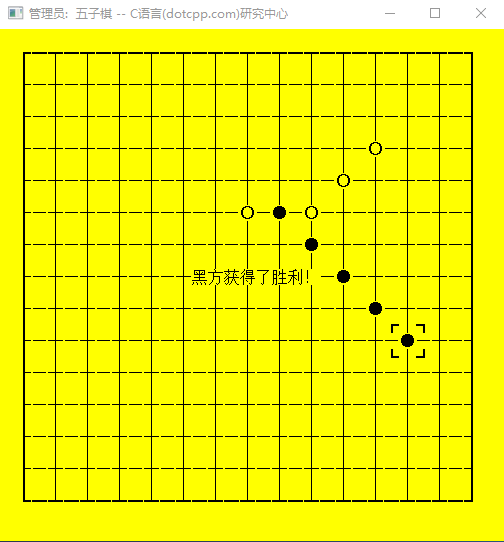• 欢迎访问C语言网www.dotcpp.com 比赛栏每月有奖月赛！举办比赛联系QQ：2045302297
• 问题反馈、粉丝交流 QQ群327452739 蓝桥杯训练群：113766799 申请群时请备注排名里的昵称
• C语言研究中心 为您提供有图、有料、解渴的C语言专题！ 欢迎讨论！

C语言版五子棋游戏（VC6.0实现）

25082次浏览

C语言实现五子棋游戏，编译运行后如图#include <stdlib.h>
#include <stdio.h>
#include <conio.h>
#include <string.h>
#define MAXIMUS 15 //定义棋盘大小
int p[MAXIMUS][MAXIMUS];//存储对局信息
char buff[MAXIMUS*2+1][MAXIMUS*4+3];//输出缓冲器
int Cx,Cy;//当前光标位置
int Now;//当前走子的玩家，1代表黑，2代表白
int wl,wp;//当前写入缓冲器的列数和行数位置
char* showText;//在棋盘中央显示的文字信息
int count;//回合数
char* Copy(char* strDest,const char* strSrc)//修改过的字符串复制函数，会忽略末端的\0
{
char* strDestCopy = strDest;
while (*strSrc!='\0')
{
*strDest++=*strSrc++;
}
return strDestCopy;
}
void Initialize()//初始化一个对局函数
{
int i,j;//循环变量
showText="";//重置显示信息
count=0;//回合数归零
for(i=0;i<MAXIMUS;i++)//重置对局数据
{
for(j=0;j<MAXIMUS;j++)
{
p[i][j]=0;
}
}
Cx=Cy=MAXIMUS/2;//重置光标到中央
Now=1;//重置当前为黑方
}
char* getStyle(int i,int j)//获得棋盘中指定坐标交点位置的字符，通过制表符拼成棋盘
{
if(p[i][j]==1)//1为黑子
return "●";
else if(p[i][j]==2)//2为白子
return "○";
else if(i==0&&j==0)//以下为边缘棋盘样式
return "┏";
else if(i==MAXIMUS-1&&j==0)
return "┓";
else if(i==MAXIMUS-1&&j==MAXIMUS-1)
return "┛";
else if(i==0&&j==MAXIMUS-1)
return "┗";
else if(i==0)
return "┠";
else if(i==MAXIMUS-1)
return "┨";
else if(j==0)
return "┯";
else if(j==MAXIMUS-1)
return "┷";
return "┼";//中间的空位
}
char* getCurse(int i,int j)
{//获得指定坐标交点位置左上格的样式，通过制表符来模拟光标的显示
if(i==Cx)
{
if(j==Cy)
return "┏";
else if (j==Cy+1)
return "┗";
}
else if(i==Cx+1)
{
if(j==Cy)
return "┓";
else if (j==Cy+1)
return "┛";
}
return "　";//如果不在光标附近则为空
}
void write(char* c)//向缓冲器写入字符串
{
Copy(buff[wl]+wp,c);
wp+=strlen(c);
}
void ln()//缓冲器写入位置提行
{
wl+=1;
wp=0;
}
void Display()//将缓冲器内容输出到屏幕
{
int i,l=strlen(showText);//循环变量，中间文字信息的长度
int Offset=MAXIMUS*2+2-l/2;//算出中间文字信息居中显示所在的横坐标位置
if(Offset%2==1)//如果位置为奇数，则移动到偶数，避免混乱
{
Offset--;
}
Copy(buff[MAXIMUS]+Offset,showText);//讲中间文字信息复制到缓冲器
if(l%2==1)//如果中间文字长度为半角奇数，则补上空格，避免混乱
{
*(buff[MAXIMUS]+Offset+l)=0x20;
}
system("cls");//清理屏幕，准备写入
for(i=0;i<MAXIMUS*2+1;i++){//循环写入每一行
printf("%s",buff[i]);
if(i<MAXIMUS*2)//写入完每一行需要换行
printf("\n");
}
}
void Print()//将整个棋盘算出并储存到缓冲器，然后调用Display函数显示出来
{
int i,j;//循环变量
wl=0;
wp=0;
for(j=0;j<=MAXIMUS;j++)//写入出交点左上角的字符，因为需要打印棋盘右下角，所以很以横纵各多一次循环
{
for(i=0;i<=MAXIMUS;i++)
{
write(getCurse(i,j));//写入左上角字符
if(j==0||j==MAXIMUS)//如果是棋上下盘边缘则没有连接的竖线，用空格填充位置
{
if(i!=MAXIMUS)
write("　");
}
else//如果在棋盘中间则用竖线承接上下
{
if(i==0||i==MAXIMUS-1)//左右边缘的竖线更粗
write("┃");
else if(i!=MAXIMUS)//中间的竖线
write("│");
}
}
if(j==MAXIMUS)//如果是最后一次循环，则只需要处理边侧字符，交点要少一排
{
break;
}
ln();//提行开始打印交点内容
write("　");//用空位补齐位置
for(i=0;i<MAXIMUS;i++)//按横坐标循环正常的次数
{
write(getStyle(i,j));//写入交点字符
if(i!=MAXIMUS-1)//如果不在最右侧则补充一个横线承接左右
{
if(j==0||j==MAXIMUS-1)
{
write("━");//上下边缘的横线更粗
}
else
{
write("—");//中间的横线
}
}
}
ln();//写完一行后提行
}
Display();//将缓冲器内容输出到屏幕
}
int Put(){//在当前光标位置走子，如果非空，则返回0表示失败
if(p[Cx][Cy]==0)
{
p[Cx][Cy]=Now;//改变该位置数据
return 1;//返回1表示成功
}
else
{
return 0;
}
}
int Check()//胜负检查，即判断当前走子位置有没有造成五连珠的情况
{
int w=1,x=1,y=1,z=1,i;//累计横竖正斜反邪四个方向的连续相同棋子数目
for(i=1;i<5;i++)if(Cy+i<MAXIMUS&&p[Cx][Cy+i]==Now)w++;else break;//向下检查
for(i=1;i<5;i++)if(Cy-i>0&&p[Cx][Cy-i]==Now)w++;else break;//向上检查
if(w>=5)return Now;//若果达到5个则判断当前走子玩家为赢家
for(i=1;i<5;i++)if(Cx+i<MAXIMUS&&p[Cx+i][Cy]==Now)x++;else break;//向右检查
for(i=1;i<5;i++)if(Cx-i>0&&p[Cx-i][Cy]==Now)x++;else break;//向左检查
if(x>=5)return Now;//若果达到5个则判断当前走子玩家为赢家
for(i=1;i<5;i++)if(Cx+i<MAXIMUS&&Cy+i<MAXIMUS&&p[Cx+i][Cy+i]==Now)y++;else break;//向右下检查
for(i=1;i<5;i++)if(Cx-i>0&&Cy-i>0&&p[Cx-i][Cy-i]==Now)y++;else break;//向左上检查
if(y>=5)return Now;//若果达到5个则判断当前走子玩家为赢家
for(i=1;i<5;i++)if(Cx+i<MAXIMUS&&Cy-i>0&&p[Cx+i][Cy-i]==Now)z++;else break;//向右上检查
for(i=1;i<5;i++)if(Cx-i>0&&Cy+i<MAXIMUS&&p[Cx-i][Cy+i]==Now)z++;else break;//向左下检查 if(z>=5)return Now;//若果达到5个则判断当前走子玩家为赢家
return 0;//若没有检查到五连珠，则返回0表示还没有玩家达成胜利
}
int RunGame()//进行整个对局，返回赢家信息(虽然有用上)
{
int input;//输入变量
int victor;//赢家信息
Initialize();//初始化对局
while(1){//开始无限回合的死循环，直到出现胜利跳出
Print();//打印棋盘
input=getch();//等待键盘按下一个字符
if(input==27)//如果是ESC则退出程序
{
exit(0);
}
else if(input==0x20)//如果是空格则开始走子
{
if(Put())//如果走子成功则判断胜负
{
victor=Check();
Now=3-Now;//轮换当前走子玩家
count++;
if(victor==1)//如果黑方达到胜利，显示提示文字并等待一次按键，返回胜利信息
{
showText="黑方获得了胜利！";
Print();
if(getch()==0xE0)
{
getch();
}
return Now;
}
else if(victor==2)//如果白方达到胜利，显示提示文字并等待一次按键，返回胜利信息
{
showText="白方获得了胜利！";
Display();
if(getch()==0xE0)
{
getch();
}
return Now;
}else if(count==MAXIMUS*MAXIMUS)//如果回合数达到了棋盘总量，即棋盘充满，即为平局
{
showText="平局！";
Display();
if(getch()==0xE0)
{
getch();
}
return 0;
}
}
}
else if(input==0xE0)//如果按下的是方向键，会填充两次输入，第一次为0xE0表示按下的是控制键
{
input=getch();//获得第二次输入信息
switch(input)//判断方向键方向并移动光标位置
{
case 0x4B:Cx--; break;
case 0x48:Cy--;break;
case 0x4D:Cx++;break;
case 0x50:Cy++;break;
}
if(Cx<0)Cx=MAXIMUS-1;//如果光标位置越界则移动到对侧
if(Cy<0)Cy=MAXIMUS-1; if(Cx>MAXIMUS-1)Cx=0;
if(Cy>MAXIMUS-1)Cy=0;
}
}
}
int main()//主函数
{
system("title 五-子-棋 -- c语言(dotcpp.com)研究中心");//设置标题
system("mode con cols=63 lines=32");//设置窗口大小
system("color E0");//设置颜色
while(1)
{//循环执行游戏
RunGame();
}
}

C语言研究中心(www.dotcpp.com)

C语言网提供「C语言、C++、算法竞赛」在线课程，全部由资深研发工程师或ACM金牌大佬亲授课，更科学、全面的课程体系，以在线视频+在线评测的学习模式学习，学练同步，拒绝理论派，真正学会编程！还有奖学金等增值福利等你！

C语言网, 版权所有丨如未注明 , 均为原创丨本网站采用BY-NC-SA协议进行授权 , 转载请注明C语言版五子棋游戏（VC6.0实现）

[jinyangH@aliyun.com](9)个小伙伴在吐槽
1. 胜负检查的右上左下判断好像不起作用。
鸽子2016-10-20 16:26 回复
• 我想说这些代码放在哪都是红的怎么运行啊
啦啦啦2019-04-25 13:52 回复
2. 貌似点击什么键都没有反应哦？
草木年华2016-10-21 10:56 回复
3. 斜方向 有bug 个人水平还不够，你们自己改吧
青村离奇2016-12-15 20:27 回复
• 求指教
学子2016-12-16 15:25 回复
• 第181行后面少一行if(z>=5)return Now;
pileilei2019-06-13 19:39 回复
4. 用Code::Blocks编译，棋盘错位，棋格不能正常显示。
C_Solver2018-02-02 16:05 回复
• 用VS2017也是一样的错误！！！
C_Solver2018-02-02 16:05 回复
5. 你这个表格有问题啊
局中人2020-07-14 06:37 回复